# Numbers - math word problems

#### Number of problems found: 1462

• DecomposeDecompose into primes and find the smallest common multiple n of (16,20) and the largest common divisor D of the pair of numbers (140,100)
• Three-digit numbersHow many three-digit numbers are from the numbers 0 2 4 6 8 (with/without repetition)?
• To improve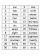To improve her handwriting Paula practices writing the numbers 1 to 200 in words. How many times will she have written the word "one" in all?
• Denominator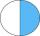Calculate the missing denominator x: ?
• Divisibility 2How many divisors has integer number 13?
• Z9–I–1In all nine fields of given shape to be filled natural numbers so that: • each of the numbers 2, 4, 6, and 8 is used at least once, • four of the inner square boxes containing the products of the numbers of adjacent cells of the outer square, • in the cir
• ABS CNCalculate the absolute value of complex number -15-29i.
• Complex number coordinatesWhich coordinates show the location of -2+3i
• DigitsWrite the smallest and largest 2-digit number.
• One sixth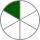How many sixths are two thirds?
• Six-digit primesFind all six-digit prime numbers that contain each one of digits 1,2,4,5,7 and 8 just once. How many are they?
• Is equal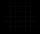Is equal following terms? ?
• Expanded formWhat is the expanded form of 0.21?
• DigitsShow that if x, y, z are 3 consecutive nonzero digits, zyx-xyz = 198, where zyx and xyz are three-digit numbers created from x, y, z.
• Linear imaginary equationGiven that ? "this is z star" Find the value of the complex number z.
• ReciprocalCalculate reciprocal of z=0.8-1.8i:
• RatioIncrease in the ratio 20:4 number 18.5.
• The modulus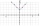Find the modulus of the complex number 2 + 5i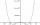By introducing a new variable solve biquadratic equation: ?Jana and David train the addition of the decimal numbers so that each of them will write a single number and these two numbers then add up. The last example was 11.11. David's number had the same number of digits before the decimal point, the Jane's numbe